# Number Tracing Worksheets

Number Tracing Worksheets for Kindergarten students to learn how to trace numbers. They will be able to trace the numbers from 1 to 20, one number per sheet.

Showing 1-20 of 20 records

•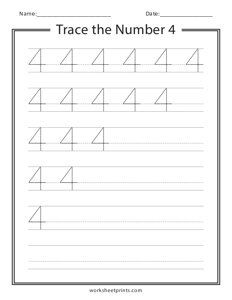Trace the Number 4

sheets, sheet, tracing, numbers

•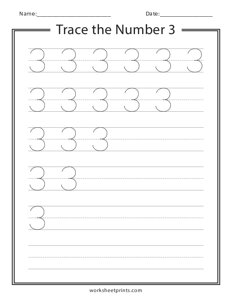Trace the Number 3

sheets, sheet, tracing, numbers

•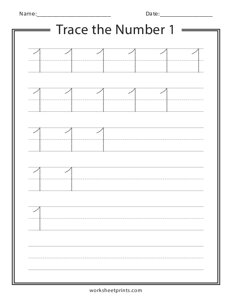Trace the Number 1

sheets, sheet, tracing, numbers

•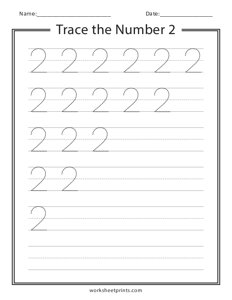Trace the Number 2

sheets, sheet, tracing, numbers

•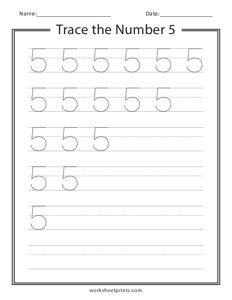Trace the Number 5

sheets, sheet, tracing, numbers

•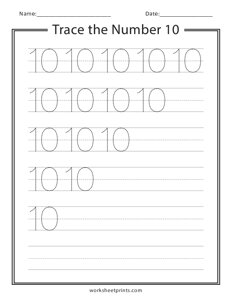Trace the Number 10

sheets, sheet, tracing, numbers

•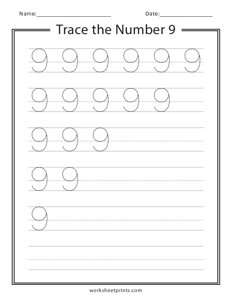Trace the Number 9

sheets, sheet, tracing, numbers

•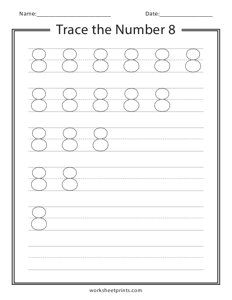Trace the Number 8

sheets, sheet, tracing, numbers

•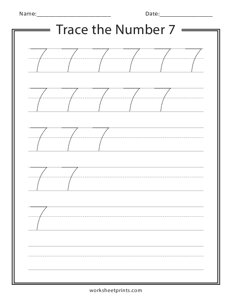Trace the Number 7

sheets, sheet, tracing, numbers

•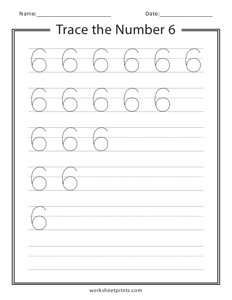Trace the Number 6

sheets, sheet, tracing, numbers

•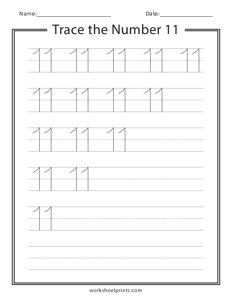Trace the Number 11

sheets, sheet, tracing, numbers

•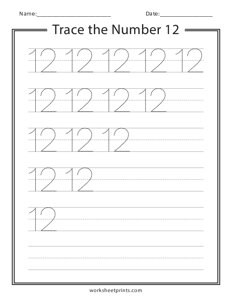Trace the Number 12

sheets, sheet, tracing, numbers

•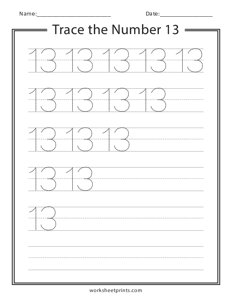Trace the Number 13

sheets, sheet, tracing, numbers

•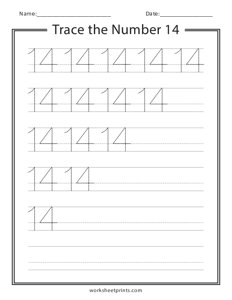Trace the Number 14

sheets, sheet, tracing, numbers

•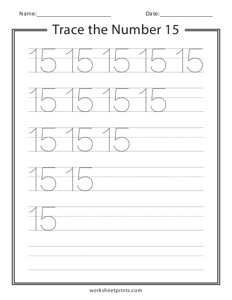Trace the Number 15

sheets, sheet, tracing, numbers

•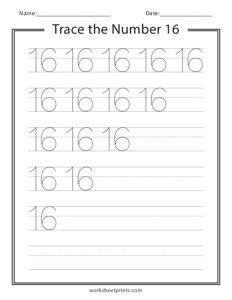Trace the Number 16

sheets, sheet, tracing, numbers

•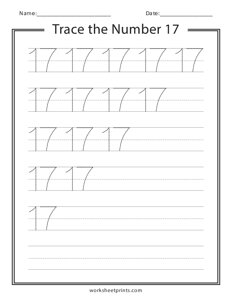Trace the Number 17

sheets, sheet, tracing, numbers

•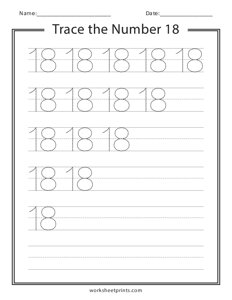Trace the Number 18

sheets, sheet, tracing, numbers

•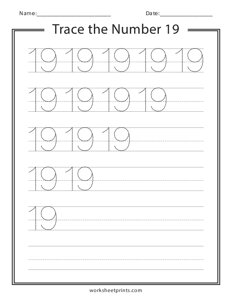Trace the Number 19

sheets, sheet, tracing, numbers

•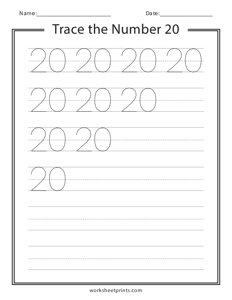Trace the Number 20

sheets, sheet, tracing, numbers